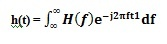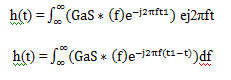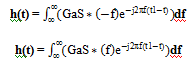# What is Matched Filter : Working & Its Applications

A filter in signal processing is an electronic circuit that allows certain frequencies while filtering out other frequencies. Therefore, a filter can remove the intended frequencies from different signals that also include irrelevant or undesirable frequencies. In the electronics field, a filter is used in different practical applications like audio electronics, radio communications, DC power supplies, ADC, etc. There are different kinds of filters available like an active filter, bandpass filter, passive filter, low pass filter, matched filter, high pass filter, bandstop filter. Each filter has its own function and application. So this article discusses an overview of one of the types of filter namely matched filter – working & its applications.

## What is Matched Filter?

Matched filter definition is: if a filter generates an output to maximize the output peak power ratio to mean noise power within its frequency response then it is called a matched filter. In telecommunications, it is the optimal linear filter used to increase the SNR or signal-to-noise ratio in the existence of additive stochastic noise.

These types of filters are generally used in radar, where a known signal is transmitted out & the reflected signal can be compared with the transmitted signal. The best example of the matched filter is pulse compression because the impulse response can be matched with input pulse signals. In image processing, two dimensional matched filters are used to enhance the X-Ray or SNR observations

### Characteristics

The characteristics of matched filter include the following.

• The Signal to noise ratio maximization is possible even for non-Gaussian noise.
• The output generated by this filter is like signal energy in the nonexistence of noise.
• They are applicable for the detection of signals.

### Matched Filter Block Diagram

The block diagram of the matched filter is shown below. Consider the following diagram where g(t) is the input signal & w(t) is the white noise. These two signals are fed to the h(t) filter, which maximizes the signal-to-noise ratio(SNR) of the y(t) output.

The input of filter ‘x(t)’ includes a pulse signal ‘g(t) that is corrupted through additive channel noise ‘w(t)’ which is shown in the following.

X(t) = g(t) + w(t)

Where ‘t’ is an arbitrary observation interval. g(t) is the pulse signal that may signify a with a binary symbol like 0 or 1 within a digital communication system. The ‘w(t)’ is the sample function of a white noise procedure of zero mean & power spectral density is No/2. The source of uncertainty mainly lies in the noise.

The receiver shown in the  diagram should be able to receive the pulse signal g(t) with a good SNR, so the output can be given as y(t) that is sampled at t =T.

So this requirement can be satisfied by optimizing the filter design to reduce the noise effects at the output of the filter in some numerical sense, and thus improve the pulse detection of the signal g(t). Since this filter is linear, then the output ‘y(t)’ may be simply expressed as

y(t) = g0(t) + n(t)

From the above equation, go(t) is the linear output corresponding to the pulse signal g(t) & n(t) is filtered noise. Both the go(t) and n(t) are generated by the signal & the noise components of the input x(t) correspondingly. The purpose of the matched filter is to maximise the output signal-to-noise ratio.

Here, the matched filter is a type of linear filter having an impulse response h(t) in the time domain, and in frequency response, is denoted by H(f) and when signal go(t) sampled at  t= T >> the average power of the filter noise. Then the maximum signal to noise ratio denoted by ‘Ƞ’ would be

Ƞ = |go(T) |2 / E[n^2 (t)]

Where,

|go(T) |2 is instantaneous power in the output signal.

E is the statistical expectation operator.

E[n^2 (t)] is the average output noise power.

#### Matched Filter Working

The working of a matched filter in signal processing is done by comparing a recognized template or delayed signal with an unidentified signal to notice the existence of the template within the unknown signal. So this is analogous to convolving the unidentified signal throughout a conjugated time-reversed template version.

### Matched Filter Equation Derivation

The derivations of matched filter mainly include frequency response and an impulse response which are discussed below.

#### Frequency Response of Matched Filter

The Matched filter’s frequency response is proportional to the complexity of the spectrum of input signals. So the expression for matched filer frequency response can be mathematically written as

H(f) = GaS* (f) e^-j2πft1

Where,

‘Ga’ is the filter’s maximum gain.

S(f) = Input signal’s fourier transform

S*(f) = Complex conjugate of S(f)

‘t1’ is the instant time where the observed signal is maximum.

Generally, the value of ‘Ga’ is considered as ‘1’, so the equation will become as

H(f) = S* (f) e^-j2πft1

The matched filter frequency response equation will have the magnitude of S∗(f) & phase angle of e^-j2πft1, which changes through frequency consistently.

#### Impulse Response of Matched Filter

In the time domain, the output of filter receiver ‘h(t)’ can be obtained through applying the IFT or inverse Fourier transform of the frequency response function, H(f).We know the equation of H(f) = GaS* (f) e^-j2πft1, substitute this in the above equation.We know the relation S*(f) = S*(-f)

Substitute the above equation in the ‘h(t)’ equation.h(t) = GaS (t1-t)

Generally, the ‘Ga’ value can be considered as ‘1’ then the equation will become as

h(t) = S (t1-t)

The equation above proves that the Matched filter’s impulse response ‘h(t)’ is the received signal’s ‘s(t)’ mirror image about a time instant ‘ t1’.

#### Matched Filter Detector

The main purpose of a matched-filter detector is to identify the occurrence of an MPSK signal in white Gaussian noise. The matched filter’s detection of false alarms and detection probabilities are estimated through Craig’s method.

This detector can be evaluated with the optimal Bayesian detector because the Bayesian detector’s performance is simply an upper bound for the performance of detection. So, the matched filter’s detection rules, as well as the optimal detector, are not similar, but their performances are the same.

It is also revealed that the conventional energy detector is significantly suboptimal for the MPSK signal’s activity detection. An energy detector that is modified can be proposed depending on both the performances of the Bayesian detectors & matched filter’s decision regions.

So, the performance of matched filter detector is very close to the optimal detector, although its implementation needs very low computational complexity.

### Different Types of Matched Filter

Matched filters are classified into two types two dimensional (2D) and three dimensional (3D) matched filters which are discussed below.

#### 2D Matched Filter

The two-dimensional matched filter or 2D matched filter is used in SAR (Synthetic aperture radar) to enhance the SNR or signal to noise ratio however the gain of SNR of synthetic aperture radar is controversial. The traditional technique examines the signal-to-noise ratio of SAR based on coherent integration & pulse compression; however, it will have diverse calculation results.

This type of filter is mainly used in image processing for enhancing the signal-to-noise ratio of X-ray observations. This filtering is a demodulation method through linear time-invariant filters to enhance SNR.

#### 3D Matched Filter

The 3D matched filter is a powerful processing technique used to detect moving, weak & optical targets that are immersed in a noise field. This process uses the 3D processor to process the whole series of optical scene frames that include moving objects. So this processor must be matched properly to the object signature & its velocity vector, however, it will detect all objects simultaneously to which it is matched.

The simulation results will show the capacity of the processor to detect objects well under the level of background. These results will also show some effects on several processed frames, mismatch of velocity & signature.

The advantages of matched filter are shown below.

• This filter enhances the signal-to-noise ratio by decreasing the spectral bandwidth of noise & additionally it decreases the noise in the bandwidth wavelet through the wavelet spectrum shape.
• This filter does not conserve the input signal shape and the output of this filter is the transmitted signal’s autocorrelation function.
• They have the capacity to maintain axial resolution through depth, depth of tissue penetration & increased sensitivity.
• This filter is very efficient once the detected signal waveform is known perfectly & when the interference present is only white noise.
• This filter has optimal performance & its computational cost is low.
• It needs fewer signal samples to obtain good detection.

The disadvantages of matched filter are shown below.

• The matched filter’s main disadvantage within two dimensions is its sensitivity toward object rotation, shifts & scaling particularly in the additive colored Gaussian noise existence for unknown covariance.
• It needs past information of the primary user.
• It consumes more power.
• It has high complexity.

### Applications

The applications of matched filters are shown below.

• The application of matched filter is Radar imaging.
• Matched-filtering is an optimum detection technique used to remove a transmitted signal which is monitored throughout the noise signal.
• This filter is used for signal detection in white noise & linear channel conditions.
• This filter is used to match a specific transit waveform within communications.
• This type of filter maximizes the signal-to-noise ratio.
• A 2-dimensional matched filter is used to place target patterns within images.
• These filters are frequently used in signal detection for comparing a known signal through an unknown signal to notice the existence of the template within the unknown signal.
• This filter is used in a wide range of applications that involve pattern recognition like detection of an image, radar signal interpretation & frequency shift keying demodulation.

Thus, this is all about an overview of a Matched filter which includes its types, working, advantages, disadvantages, and applications. This filter is used to extract known wavelets from a polluted signal. This filter will maximize the SNR of the signal which is detected with respect to the noise. Here is a question for you, who invented matched filter?